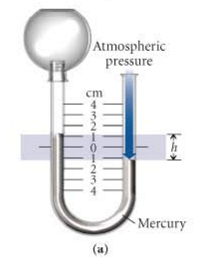Problem: In diagram a, what is the pressure in the manometer if h = 2.00 cm and the barometric pressure is 780.0 mmHg? a) 780.0 mmHg b) 778 mmHg c) 1.00 atm  d) 1.02 atm e) 782 mmHg

FREE Expert Solution
98% (163 ratings)
Problem Details

In diagram a, what is the pressure in the manometer if h = 2.00 cm and the barometric pressure is 780.0 mmHg?

a) 780.0 mmHg

b) 778 mmHg

c) 1.00 atm

d) 1.02 atm

e) 782 mmHgWhat scientific concept do you need to know in order to solve this problem?

Our tutors have indicated that to solve this problem you will need to apply the Manometer concept. If you need more Manometer practice, you can also practice Manometer practice problems .

What is the difficulty of this problem?

Our tutors rated the difficulty of In diagram a, what is the pressure in the manometer if h = 2... as medium difficulty.

How long does this problem take to solve?

Our expert Chemistry tutor, Jules took 2 minutes to solve this problem. You can follow their steps in the video explanation above.

What professor is this problem relevant for?

Based on our data, we think this problem is relevant for Professor Zastrow's class at UH.